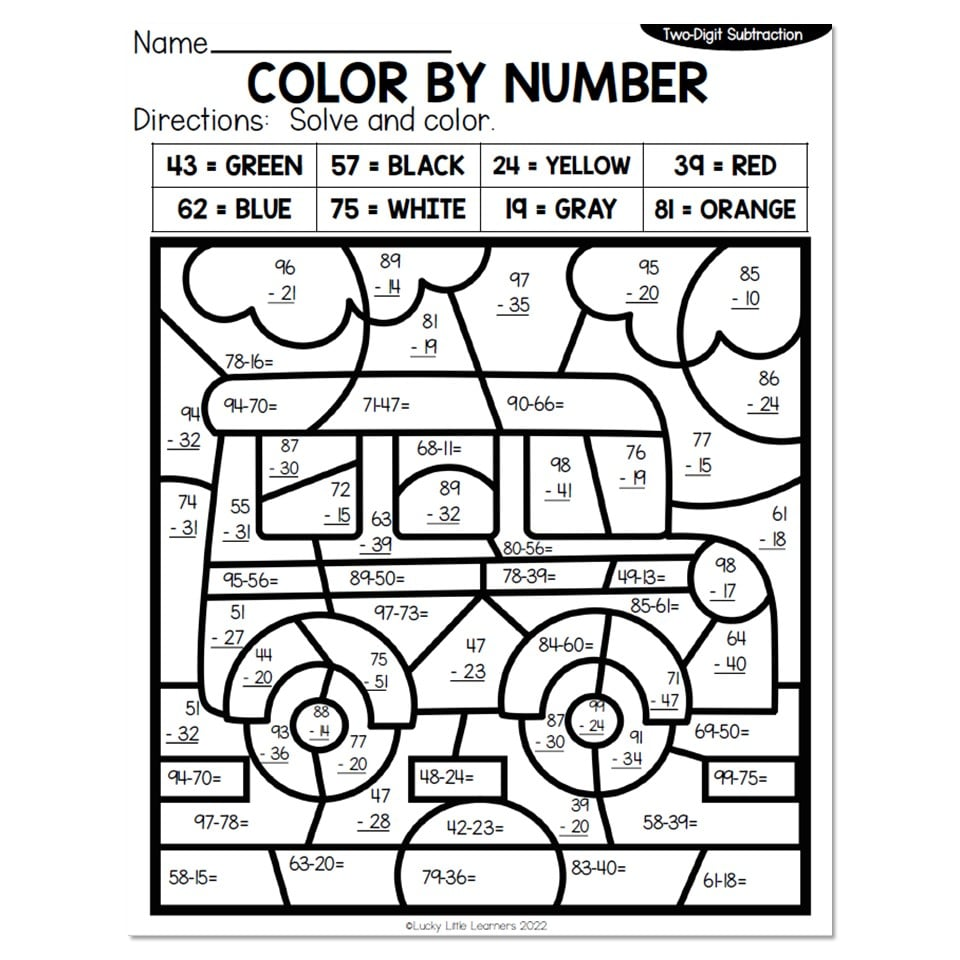Home » Blog » Operations » Subtraction » Videos that Teach Subtraction

# Videos that Teach Subtraction

Written by: Angie Olson

Introducing students to the concept of subtraction can be fun and interesting!  We have compiled a list of videos that teach subtraction that are both informative and appropriate for students.  Enjoy!

## Subtraction for Kids | Classroom Edition for 1st & 2nd Grade (8:06)

This video is from an author called Homeschool Pop that is designed for thorough classroom or at-home learning. In this subtraction math lesson video, learn basic subtraction for 1st grade and learn the foundation for subtraction for 2nd grade math lessons. Entertaining and cute, our animated friend learns about subtraction as he gives away his cowboy hats to his friends!

## Subtraction Song – My Dog Subtraction (4:08)

This is a song about a dog whose name is subtraction and shows us the basics of subtractions as candles are disappearing from a birthday cake. This video turns word problems into equations and is great for beginners’ understanding of taking away!

## When You Subtract with a Pirate (4:30)

This fun pirate-themed video shows students how to subtract as the grumpy pirate narrator makes people walk the plank. The equations are easy to understand and the tag line in the song is very catchy, perfect for subtraction review!

## Basic Subtraction Math Lesson – Learn to Subtract (1:49)

This short but sweet video is great for beginners because of its clarity, repetition, and practice of subtraction problems. The narrator begins each problem with “if we have __ then take away __ we have __left”, which is perfect for teaching the understanding of the basic principles of subtraction.

## Subtraction Facts All Mixed Up 1- Jack Hartmann (4:38)

In this video, Jack Hartmann gives students the opportunity to practice subtraction facts all mixed up to help review and sharpen their skills. The first time through he gives the subtraction fact with the answer, showing a visual of the problem on ten frames. The second time through the delays the answer for students to answer before the answer appears, and the last time students answer all on their own.

## Subtraction Song- The Mystery of the Chocolate Donuts (4:11)

How did the boy end up with chocolate donuts? Find out in this musical video that teaches students how to subtract while exposing them to several different methods of subtraction, such as counting back, taking away, and math equations.

## Subtract by Counting Back (2:27)

This is a simple video that is a good reinforcer if you have students who are having trouble with the concept. It is clear, visual, and easy to understand as the instructor walks you through crossing out the objects and building subtraction equastions by counting back.

## Subtraction with the Help of Stories (3:44)

This video is very helpful for introducing the concept of taking word problems (or stories) and turning them into subtraction equations. There are three stories where the narrator walks us through how to understand math in everyday life.

## How to Borrow in Subtraction! …for Kids!(6:40)

This hilarious video is a perfect introduction to borrowing in subtraction. The puppets with goofy accents, Steve and Andy, walk us through how to borrow in subtraction with a pizza store analogy that is educational, engaging, and outright silly!

Use the 2-Digit Subtraction With and Without Regrouping Color by Number sheets to get some fun practice in!## Lucky to Learn Math Subtraction Videos

Each Lucky to Learn math unit lesson features a Warm-Up Activity to get students in a math mindset. The integrated theme for Unit 3 is all about factories, production, and manufacturing. Students will explore a candy factory, learn how books are made, and see a pizza assembly line, all from the comfort of their classroom! Students will make real-life connections to understand how and why using addition and subtraction strategies is essential to this business! You can find a selection of videos that will get students thinking about addition strategies in a whole new way!

## More Math Videos

Need some more teacher-approved videos to add to your instruction? Take a look at the list below.